# Python For Trading: An Introduction

By Vibhu Singh, Shagufta Tahsildar, and Rekhit Pachanekar

Python, a programming language which was conceived in the late 1980s by Guido Van Rossum, has witnessed humongous growth, especially in the recent years due to its ease of use, extensive libraries, and elegant syntax.

How did a programming language land up with a name like ‘Python’? Well, Guido, the creator of Python, needed a short, unique, and a slightly mysterious name and thus decided on “Python” while watching a comedy series called “Monty Python’s Flying Circus”.

If you are curious on knowing the history of Python as well as what is Python and its applications, you can always refer to the first chapter of the Python Handbook, which serves as your guide as you start your journey in Python.

We are moving towards the world of automation and thus, there is always a demand for people with a programming language experience. When it comes to the world of algorithmic trading, it is necessary to learn a programming language in order to make your trading algorithms smarter as well as faster. It is true that you can outsource the coding part of your strategy to a competent programmer but it will be cumbersome later when you have to tweak your strategy according to the changing market scenario.

## Why Python?

Before we understand the core concepts of Python and its application in finance as well as Python trading, let us understand the reason we should learn Python.

Having knowledge of a popular programming language is the building block to becoming a professional algorithmic trader. With rapid advancements in technology every day- it is difficult for programmers to learn all the programming languages. One of the most common questions that we receive at QuantInsti is “Which programming language should I learn for algorithmic trading?” The answer to this question is that there is nothing like a “BEST” language for algorithmic trading. There are many important concepts taken into consideration in the entire trading process before choosing a programming language - cost, performance, resiliency, modularity and various other trading strategy parameters.

Each programming language has its own pros and cons and a balance between the pros and cons based on the requirements of the trading system will affect the choice of programming language an individual might prefer to learn. Every organization has a different programming language based on their business and culture.

●    What kind of trading system will you use?
●    Are you planning to design an execution based trading system?
●    Are you in need of a high-performance backtester?

Based on the answers to all these questions, one can decide on which programming language is the best for algorithmic trading. However, to answer the above questions let’s explore the various programming languages used for algorithmic trading with a brief understanding of the pros and cons of each.

Quant traders require a scripting language to build a prototype of the code. In that regard, Python has a huge significance in the overall trading process as it finds applications in prototyping quant models particularly in quant trading groups in banks and hedge funds. Most of the quant traders prefer Python trading as it helps them build their own data connectors, execution mechanisms, backtesting, risk and order management, walk forward analysis and optimization testing modules.

Algorithmic trading developers are often confused whether to choose an open source technology or a commercial/proprietary technology. Before deciding on this it is important to consider the activity of the community surrounding a particular programming language, the ease of maintenance, ease of installation, documentation of the language and the maintenance costs. Python trading has become a preferred choice recently as Python is an open source and all the packages are free for commercial use.

Python trading has gained traction in the quant finance community as it makes it easy to build intricate statistical models with ease due to the availability of sufficient scientific libraries like Pandas, NumPy, PyAlgoTrade, Pybacktest and more. First updates to python trading libraries are a regular occurence in the developer community.
In fact, according to the Developer Survey Results 2019 at stackOverflow, Python is the fastest growing programming language.

It was also found that among the languages the people were most interested to learn, Python was the most desired programming language.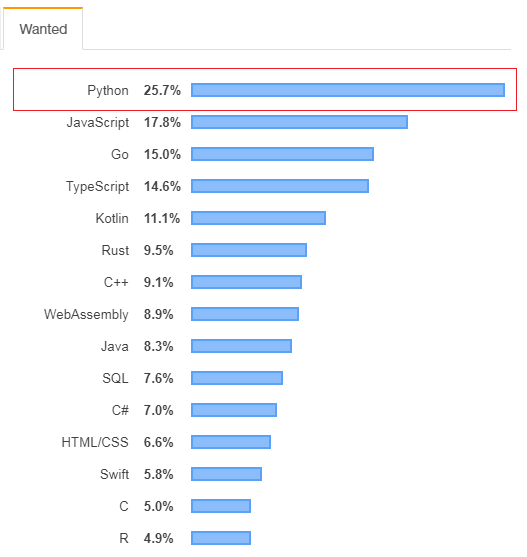## Benefits and Drawbacks of Python in Algorithmic Trading

Let us list down a few benefits of Python first.

• Parallelization and huge computational power of Python give scalability to the portfolio.
• Python makes it easier to write and evaluate algo trading structures because of its functional programming approach. The code can be easily extended to dynamic algorithms for trading.
• Python can be used to develop some great trading platforms whereas using C or C++ is a hassle and time-consuming job.
• Python trading is an ideal choice for people who want to become pioneers with dynamic algo trading platforms.
• For individuals new to algorithmic trading, the Python code is easily readable and accessible.
• It is comparatively easier to fix new modules to Python language and make it expansive.
• The existing modules also make it easier for algo traders to share functionality amongst different programs by decomposing them into individual modules which can be applied to various trading architectures.
• When using Python for trading it requires fewer lines of code due to the availability of extensive libraries.
• Quant traders can skip various steps which other languages like C or C++ might require.
• This also brings down the overall cost of maintaining the trading system.
• With a wide range of scientific libraries in Python, algorithmic traders can perform any kind of data analysis at an execution speed that is comparable to compiled languages like C++.

Just like every coin has two faces, there are some drawbacks of Python trading.

In Python, every variable is considered as an object, so every variable will store unnecessary information like size, value and reference pointer. When storing millions of variables if memory management is not done effectively, it could lead to memory leaks and performance bottlenecks.

However, for someone who is starting out in the field of programming, the pros of Python trading exceed the drawbacks making it a supreme choice of programming language for algorithmic trading platforms.

## Python vs. C++ vs. R

Python is a relatively new programming language when compared to C++ and R. However, it is found that people prefer Python due to its ease of use. Let's understand the difference between Python and C++ first.

• A compiled language like C++ is often an ideal programming language choice if the backtesting parameter dimensions are large. However, Python makes use of high-performance libraries like Pandas or NumPy for backtesting to maintain competitiveness with its compiled equivalents.
• Between the two, Python or C++, the language to be used for backtesting and research environments will be decided on the basis of the requirements of the algorithm and the available libraries. Choosing C++ or Python will depend on the trading frequency. Python language is ideal for 5-minute bars but when moving downtime sub-second time frames this might not be an ideal choice.
• If speed is a distinctive factor to compete with your competition then using C++ is a better choice than using Python for Trading.
• C++ is a complicated language, unlike Python which even beginners can easily read, write and learn.

The following is the latest study by Stackoverflow that shows Python as among the Top 4 Popular programming languages.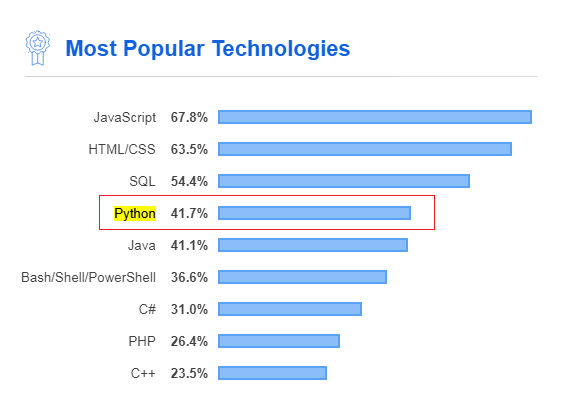We have seen above that Python is preferred to C++ in most of the situations. But what about other programming languages, like R?

Well, the answer is that you can use either based on your requirements but as a beginner Python is preferred as it is easier to grasp and has a cleaner syntax.

Python already consists of a myriad of libraries, which consists of numerous modules which can be used directly in our program without the need of writing code for the function.

Trading systems evolve with time and any programming language choices will evolve along with them. If you want to enjoy the best of both worlds in algorithmic trading i.e. benefits of a general-purpose programming language and powerful tools of the scientific stack - Python would most definitely satisfy all the criteria.

## Applications of Python in Finance

Apart from its huge applications in the field of web and software development, one of the reasons why Python is being extensively used nowadays is due to its applications in the field of machine learning, where machines are trained to learn from the historical data and act accordingly on some new data. Hence, it finds its use across various domains such as Medicine (to learn and predict diseases), Marketing(to understand and predict user behaviour) and now even in Trading (to analyze and build strategies based on financial data).

Today, finance professionals are enrolling for Python trading courses to stay relevant in today’s world of finance. Gone are the days when computer programmers and Finance professionals were in separate divisions. Companies are hiring computer engineers and train them in the world of finance as the world of algorithmic trading becomes the dominant way of trading in the world. Already 70% of the US stock exchange order volume has been done with algorithmic trading. Thus, it makes sense for Equity traders and the like to acquaint themselves with any programming language to better their own trading strategy.

## Getting started with Python and Setup

After going through the advantages of using Python, let’s understand how you can actually start using it. Let's talk about the various components of Python.

• AnacondaAnaconda is a distribution of Python, which means that it consists of all the tools and libraries required for the execution of our Python code. Downloading and installing libraries and tools individually can be a tedious task, which is why we install Anaconda as it consists of a majority of the Python packages which can be directly loaded to the IDE to use them.
• Spyder IDE - IDE or Integrated Development Environment, is a software platform where we can write and execute our codes. It basically consists of a code editor, to write codes, a compiler or interpreter to convert our code into machine-readable language and a debugger to identify any bugs or errors in your code. Spyder IDE can be used to create multiple projects of Python.
• Jupyter NotebookJupyter is an open-source application that allows us to create, write and implement codes in a more interactive format. It can be used to test small chunks of code, whereas we can use the Spyder IDE to implement bigger projects.
• CondaConda is a package management system which can be used to install, run and update libraries.
Note: Spyder IDE and Jupyter Notebook are a part of the Anaconda distribution; hence they need not be installed separately.

### Installation Guide for Python

Let us now begin with the installation process of Anaconda.

Follow the steps below to install and set up Anaconda on your Windows system:

#### Step 1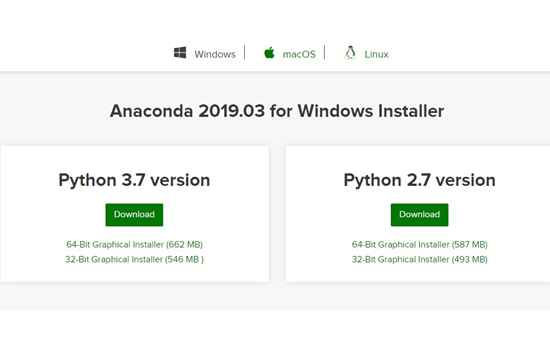#### Step 2

Run the downloaded file and click “Next” and accept the agreement by clicking “I agree”.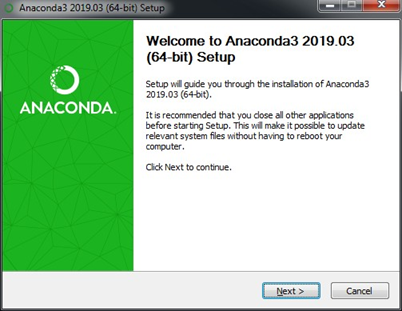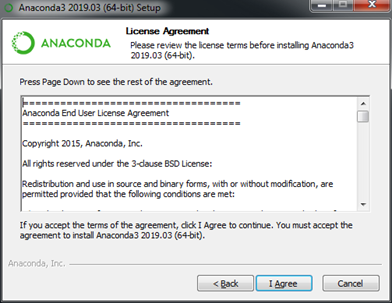#### Step 3

In select installation type, choose “Just Me (Recommended)” and choose the location where you wish to save Anaconda and click on Next.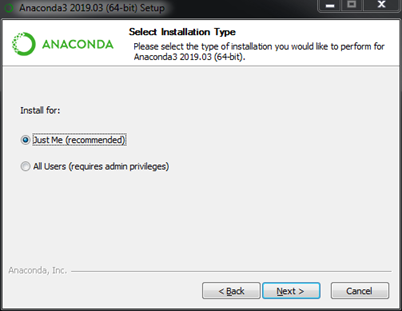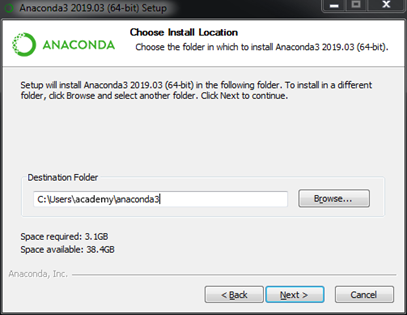Step 4
In Advanced Options, checkmark both the boxes and click on Install. Once it is installed, click “Finish”.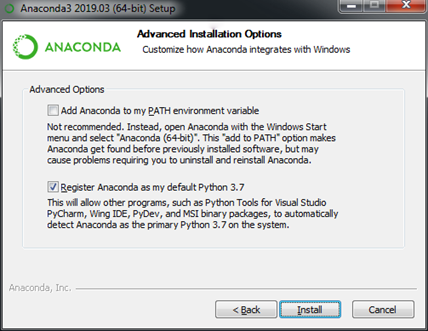Now, you have successfully installed Anaconda on your system and it is ready to run. You can open the Anaconda Navigator and find other tools like Jupyter Notebook and Spyder IDE.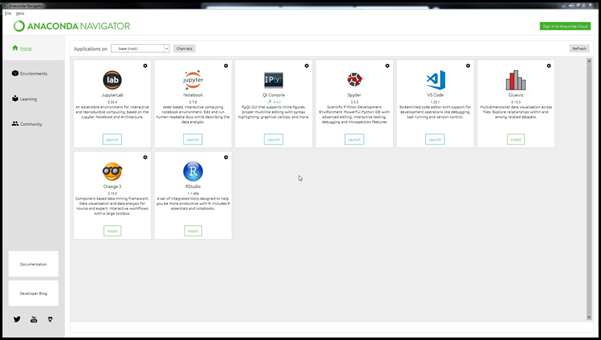Once we have installed Anaconda, we will now move on to one of the most important components of the Python landscape, i.e. Python Libraries.

Note: Anaconda provides support for Linux as well as macOS. The installation details for the OS are provided on the official website in detail.


## Popular Libraries/Packages in Python

Libraries are a collection of reusable modules or functions which can be directly used in our code to perform a certain function without the necessity to write a code for the function.

As mentioned earlier, Python has a huge collection of libraries which can be used for various functionalities like computing, machine learning, visualizations, etc. However, we will talk about the most relevant libraries required for coding trading strategies before actually getting started with Python.

We will be required to:

• import financial data,
• perform numerical analysis,
• plot graphs, and
• perform backtesting on data.

For all these functions, here are a few most widely used libraries:

• NumPyNumPy or NumericalPy, is mostly used to perform numerical computing on arrays of data. The array is an element which contains a group of elements and we can perform different operations on it using the functions of NumPy.
• PandasPandas is mostly used with DataFrame, which is a tabular or a spreadsheet format where data is stored in rows and columns. Pandas can be used to import data from Excel and CSV files directly into the Python code and perform data analysis and manipulation of the tabular data.
• MatplotlibMatplotlib is used to plot 2D graphs like bar charts, scatter plots, histograms etc. It consists of various functions to modify the graph according to our requirements too.
• TA-LibTA-Lib or Technical Analysis library is an open-source library and is extensively used to perform technical analysis on financial data using technical indicators such as RSI (Relative Strength Index), Bollinger bands, MACD etc. It not only works with Python but also with other programming languages such as C/C++, Java, Perl etc. Here are some of the functions available in  TA-Lib:
• ZiplineZipline is a Python library for trading applications that power the Quantopian service mentioned above. It is an event-driven system that supports both backtesting and live trading.

These are but a few of the libraries which you will be using as you start using Python to perfect your trading strategy.

To know about the myriad number of libraries in more detail, you can browse through this blog on Popular Python Trading platforms.

## Working with data in Python

Knowing how to retreive, format and use data is an essential part of Python trading, as without data there is nothing you can go ahead with.

Financial data is available on various online websites. This data is also called as time-series data as it is indexed by time (the timescale can be monthly, weekly, daily, 5 minutely, minutely, etc.). Apart from that, we can directly upload data from Excel sheets too which are in CSV format, which stores tabular values and can be imported to other files and codes.

Now, we will learn how to import both time-series data and data from CSV files through the examples given below.

### Importing data in Python

Here’s an example on how to import time series data from Yahoo finance along with the explanation of the command in the comments:

Note: In Python, we can add comments by adding a ‘#’ symbol at the start of the line.

To fetch data from Yahoo finance, you need to first pip install yfinance.

!pip install yfinance

# Import yfinance
import yfinance as yf

# Get the data for stock Facebook from 2017-04-01 to 2019-04-30

# Print the first five rows of the data
data.head()

Output: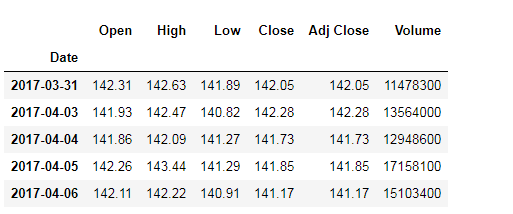Now, let’s look at another example where we can import data from an existing CSV file:

# Import pandas
import pandas as pd

# Read data from csv file
data.head()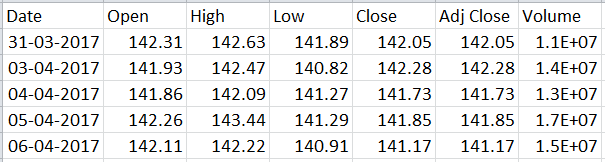## Creating a sample trading strategy and backtesting in Python

One of the simplest trading strategies involves Moving averages. But before we dive right into the coding part, we shall first discuss the mechanism on how to find different types of moving averages and then finally move on to one moving average trading strategy which is moving average convergence divergence, or in short, MACD.

### What are Moving Averages?

Moving Average also called Rolling average, is the mean or average of the specified data for a  given set of consecutive periods. As new data becomes available, the mean of the data is computed by dropping the oldest value and adding the latest one.

So, in essence, the mean or average is rolling along with the data, and hence the name ‘Moving Average’.

An example of calculating the simple moving average is as follows:
Let us assume a window of 10, ie n = 10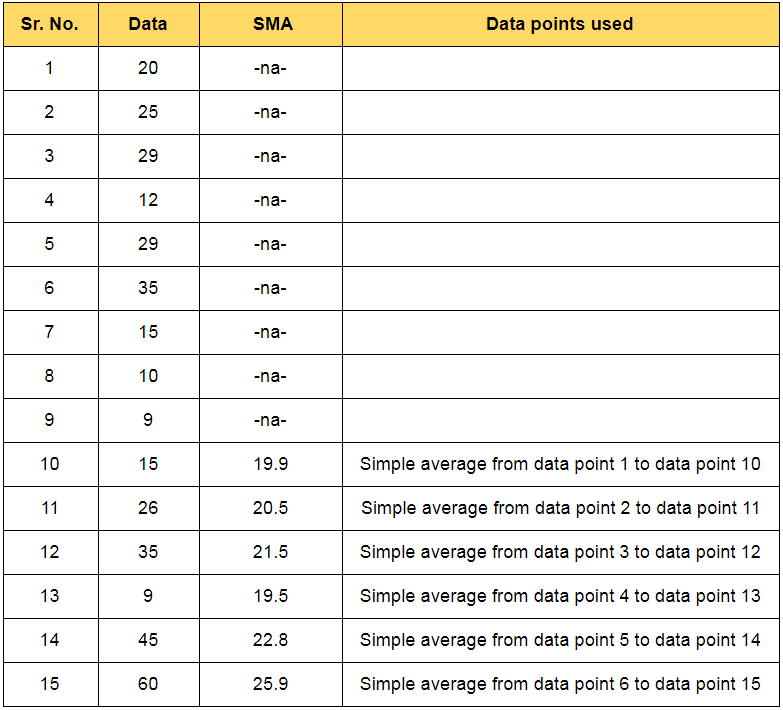In the financial market, the price of securities tends to fluctuate rapidly and as a result, when we plot the graph of the price series, it is very difficult to predict the trend or movement in the price of securities.

In such cases moving average will be helpful as it smoothens out the fluctuations, enabling traders to predict movement easily.

Slow Moving Averages: The moving averages with longer durations are known as slow-moving averages as they are slower to respond to a change in trend. This will generate smoother curves and contain lesser fluctuations.

Fast Moving Averages: The moving averages with shorter durations are known as fast-moving averages and are faster to respond to a change in trend.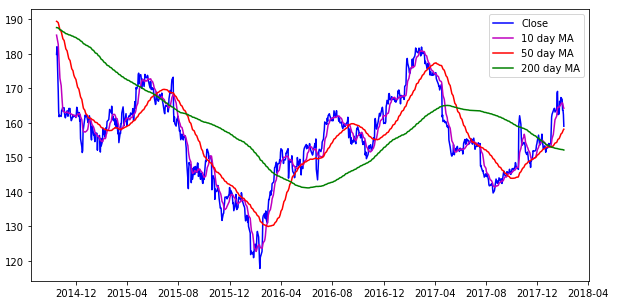Consider the chart shown above, it contains:

• the closing price of a stock IBM (blue line),
• the 10-day moving average (magnum line),
• the 50-day moving average (red line) and
• the 200-day moving average (green line).

It can be observed that the 200-day moving average is the smoothest and the 10-day moving average has the maximum number of fluctuations. Going further, you can see that the 10-day moving average line is a bit similar to the closing price graph.

### Types of Moving Averages

There are three most commonly used types of moving averages, the simple, weighted and the exponential moving average. The only noteworthy difference between the various moving averages is the weights assigned to data points in the moving average period.

Let’s understand each one in further detail:

#### Simple Moving Average (SMA)

A simple moving average (SMA) is the average price of a security over a specific period of time. The simple moving average is the simplest type of moving average and calculated by adding the elements and dividing by the number of time periods.

All elements in the SMA have the same weightage. If the moving average period is 10, then each element will have a 10% weightage in the SMA.

The formula for the simple moving average is given below:

SMA = Sum of data points in the moving average period / Total number of periods

#### Exponential Moving Average (EMA)

The logic of exponential moving average is that latest prices have more bearing on the future price than past prices. Thus, more weight is given to the current prices than to the historic prices. With the highest weight to the latest price, the weights reduce exponentially over the past prices.

This makes the exponential moving average quicker to respond to short-term price fluctuations than a simple moving average.

The formula for the exponential moving average is given below:

EMA = (Closing price - EMA*(previous day)) x multiplier  +  EMA*(previous day)

Weightage multiplier = 2 / (moving average period +1)

#### Weighted Moving Average (WMA)

The weighted moving average is the moving average resulting from the multiplication of each component with a predefined weight.

The exponential moving average is a type of weighted moving average where the elements in the moving average period are assigned an exponentially increasing weightage.

A linearly weighted moving average (LWMA), generally referred to as weighted moving average (WMA), is computed by assigning a linearly increasing weightage to the elements in the moving average period.

Now that we have an understanding of moving average and their different types, let’s try to create a trading strategy using moving average.

#### Moving Average Convergence Divergence (MACD)

Moving Average Convergence Divergence or MACD was developed by Gerald Appel in the late seventies. It is one of the simplest and effective trend-following momentum indicators.

In MACD strategy, we use two series, MACD series which is the difference between the 26-day EMA and 12-day EMA and signal series which is the 9 day EMA of MACD series.

We can trigger the trading signal using MACD series and signal series.

• When the MACD line crosses above the signal line, then it is recommended to buy the underlying security.
• When the MACD line crosses below the signal line, then a signal to sell is triggered.

#### Implementing the MACD strategy in Python

Import the necessary libraries and read the data

# Import pandas
import pandas as pd

# Import matplotlib
import matplotlib.pyplot as plt
plt.style.use('ggplot')
%matplotlib inline

data.index = pd.to_datetime(data.index, dayfirst=True)

# Visualise the data
plt.figure(figsize=(10,5))
data['Close'].plot(figsize=(10,5))
plt.legend()
plt.show()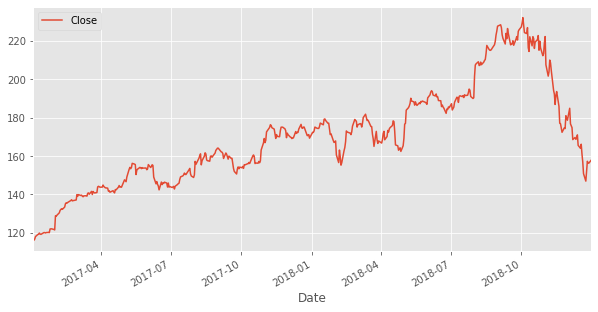Calculate and plot the MACD series which is the difference 26-day EMA and 12-day EMA and signal series which is 9 day EMA of the MACD series.

# Calculate exponential moving average

data[['Close','12d_EMA','26d_EMA']].plot(figsize=(10,5))
plt.show()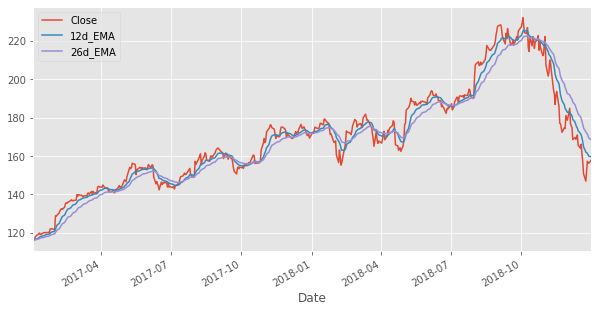# Calculate MACD
data['macd'] = data['12d_EMA']- data['26d_EMA']

# Calculate Signal

data[['macd','macdsignal']].plot(figsize=(10,5))
plt.show()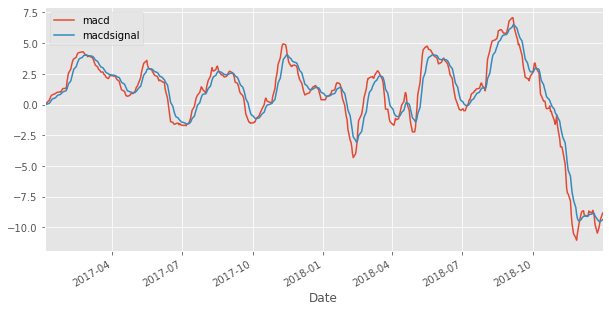When the value of MACD series is greater than signal series then buy, else sell.

# Import numpy
import numpy as np

# Define Signal
data['trading_signal'] = np.where(data['macd'] > data['macdsignal'], 1, -1)


Create and calculate the strategy return

# Calculate Returns
data['returns'] = data.Close.pct_change()

# Calculate Strategy Returns

# Calculate Cumulative Returns
cumulative_strategy_returns = (data.strategy_returns + 1).cumprod()

# Plot Strategy Returns
cumulative_strategy_returns.plot(figsize=(10,5))
plt.legend()
plt.show()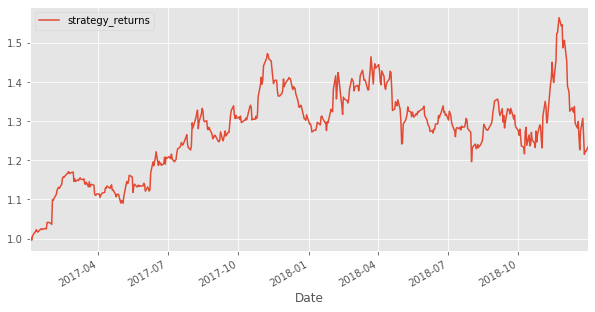## Evaluating the sample trading strategy

So far, we have created a trading strategy as well as backtested it on historical data. But does this mean it is ready to be deployed in the live markets?

Well, before we make our strategy live, we should understand its effectiveness, or in simpler words, the potential profitability of the strategy.

While there are many ways to evaluate a trading strategy, we will focus on the following,

• Annualised return,
• Annualised volatility, and
• Sharpe ratio.

Let’s understand them in detail as well as try to evaluate our own strategy based on these factors:

##### 1. Annualised Return or Compound Annual Growth Rate (CAGR)

To put it simply, CAGR is the rate of return of your investment which includes the compounding of your investment. Thus it can be used to compare two strategies and decide which one suits your needs.

Calculating CAGR
CAGR can be easily calculated with the following formula:

CAGR = [(Final value of investment /Initial value of investment)^(1/number of years)] - 1

For example, we invest in 2000 which grows to 4000 in the first year but drops to 3000 in the second year. Now, if we calculate the CAGR of the investment, it would be as follows:

CAGR =  (3000/2000)^(½) - 1 = 0.22 = 22%

For our strategy, we will try to calculate the daily returns first and then calculate the CAGR. The code, as well as the output, is given below:
In

# Total number of trading days
days = len(cumulative_strategy_returns)

# Calculate compounded annual growth rate
annual_returns = (cumulative_strategy_returns.iloc[-1]**(252/days) - 1)*100

'The CAGR is %.2f%%' % annual_returns


Out:

'The CAGR is 11.15%'


##### 2. Annualised Volatility

Before we define annualised volatility, let’s understand the meaning of volatility. A stock’s volatility is the variation in the stock price over a period of time.

For the strategy, we are using the following formula:

Annualised Volatility = square root (trading days) * square root (variance)

The code, as well as the output, is given below:
In[]

# Calculate the annualised volatility
annual_volatility = data.strategy_returns.std() * np.sqrt(252) * 100

'The annualised volatility is %.2f%%' % annual_volatility


Out []:

'The annualised volatility is 23.91%'
##### 3. Sharpe Ratio

Sharpe Ratio is basically used by investors to understand the risk taken in comparison to the risk-free investments, such as treasury bonds etc.

The sharpe ratio can be calculated in the following manner:

Sharpe ratio = [r(x) - r(f)] / δ(x)

Where,

r(x) = annualised return of investment x
r(f) = Annualised risk free rate
δ(x) = Standard deviation of r(x)

The Sharpe Ratio should be high in case of similar or peers. The code, as well as the output, is given below:
In[]

# Assume the annual risk-free rate is 6%
risk_free_rate = 0.06
daily_risk_free_return = risk_free_rate/252

# Calculate the excess returns by subtracting the daily returns by daily risk-free return
excess_daily_returns = data.strategy_returns - daily_risk_free_return

# Calculate the sharpe ratio using the given formula
sharpe_ratio = (excess_daily_returns.mean() /
excess_daily_returns.std()) * np.sqrt(252)

'The Sharpe ratio is %.2f' % sharpe_ratio


Out[]:

'The Sharpe ratio is 0.31'

## Conclusion

Python is widely used in the field of machine learning and now trading. In this article, we have covered all that would be required for getting started with Python. It is important to learn it so that you can code your own trading strategies and test them.

Its extensive libraries and modules smoothen the process of creating machine learning algorithms without the need to write huge codes.

To start learning Python and code different types of trading strategies, you can select the “Algorithmic Trading For Everyone” learning track on Quantra.

Disclaimer: All data and information provided in this article are for informational purposes only. QuantInsti® makes no representations as to accuracy, completeness, currentness, suitability, or validity of any information in this article and will not be liable for any errors, omissions, or delays in this information or any losses, injuries, or damages arising from its display or use. All information is provided on an as-is basis.